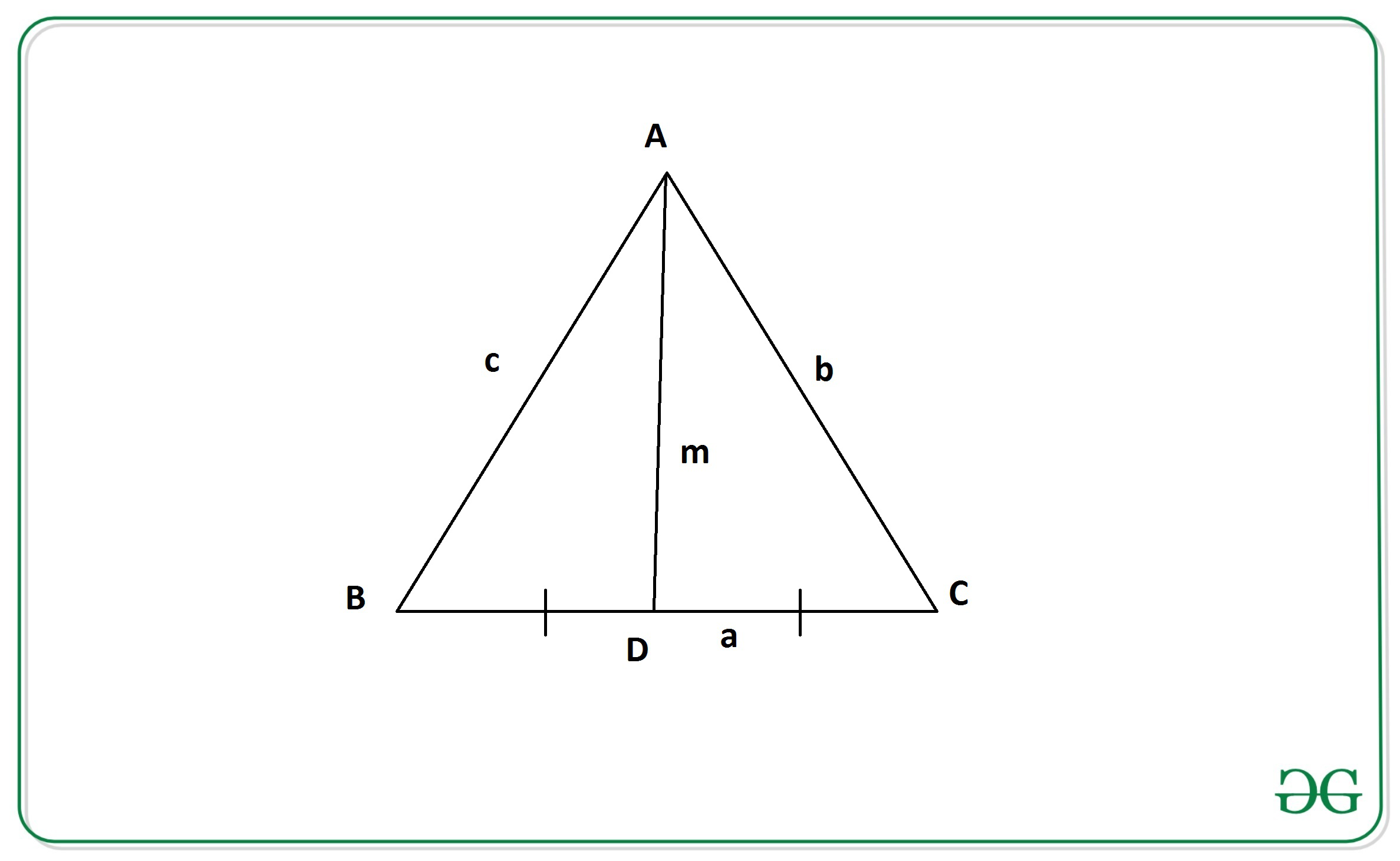# Find the length of the median of a Triangle if length of sides are given

Given the length of all three sides of a triangle as a, b and c. The task is to calculate the length of the median of the triangle.

A median of a triangle is a line segment joining a vertex to the midpoint of the opposite side, thus bisecting that side.

Examples:

Input: a = 8, b = 10, c = 13
Output: 10.89
Input: a = 4, b = 3, c = 5
Output: 3.61

Approach: The idea is to use Apollonius’s theorem to solve this problem.

Apollonius’s Theorem states that “the sum of the squares of any two sides of a triangle equals twice the square on half the third side and twice the square on the median bisecting the third side”.From the above figure, According to Apollonius’s Theorm we have:where a, b, and c are the length of sides of the triangle
and m is the length of median of the triangle on side 2*a

Therefore, the length of the median of a triangle from the above equation is given by:Below is the implementation of the above approach:

## C++

 // C++ program to find the length of the   // median using sides of the triangle  #include  using namespace std;     // Function to return the length of  // the median using sides of triangle  float median(int a, int b, int c)  {      float n = sqrt(2 * b * b +                      2 * c * c - a * a) / 2;      return n;  }     // Driver code  int main()  {      int a, b, c;      a = 4;      b = 3;      c = 5;         // Function call      float ans = median(a, b, c);          // Print final answer with 2       // digits after decimal      cout << fixed << setprecision(2) << ans;      return 0;  }     // This code is contributed by himanshu77

## Java

 // Java program to find the length of the   // median using sides of the triangle   import java.util.*;      class GFG{         // Function to return the length of   // the median using sides of triangle   public static float median(int a, int b, int c)   {       float n = (float)(Math.sqrt(2 * b * b +                                   2 * c * c -                                   a * a) / 2);       return n;   }      // Driver code  public static void main(String[] args)  {      int a, b, c;       a = 4;       b = 3;       c = 5;          // Function call       float ans = median(a, b, c);          // Print final answer with 2       // digits after decimal       System.out.println(String.format("%.2f", ans));   }  }     // This code is contributed by divyeshrabadiya07

## Python3

 # Python3 implementation to Find the   # length of the median using sides  # of the triangle     import math     # Function to return the length of   # the median using sides of triangle.   def median(a, b, c):           n = (1 / 2)*math.sqrt(2*(b**2)      + 2*(c**2)   - a**2)           return n      # Driver Code   a = 4 b = 3 c = 5    # Function Call  ans = median(a, b, c)     # Print the final answer  print(round(ans, 2))

## C#

 // C# program to find the length of the   // median using sides of the triangle   using System;     class GFG{         // Function to return the length of   // the median using sides of triangle   public static float median(int a, int b, int c)   {       float n = (float)(Math.Sqrt(2 * b * b +                                   2 * c * c -                                   a * a) / 2);       return n;   }      // Driver code  public static void Main(String[] args)  {      int a, b, c;       a = 4;       b = 3;       c = 5;          // Function call       float ans = median(a, b, c);          // Print readonly answer with 2       // digits after decimal       Console.WriteLine(String.Format("{0:F2}", ans));   }  }     // This code is contributed by gauravrajput1

Output:

3.61


Time Complexity: O(1)
Space Complexity: O(1)

Attention reader! Don’t stop learning now. Get hold of all the important DSA concepts with the DSA Self Paced Course at a student-friendly price and become industry ready.

My Personal Notes arrow_drop_upCheck out this Author's contributed articles.

If you like GeeksforGeeks and would like to contribute, you can also write an article using contribute.geeksforgeeks.org or mail your article to contribute@geeksforgeeks.org. See your article appearing on the GeeksforGeeks main page and help other Geeks.

Please Improve this article if you find anything incorrect by clicking on the "Improve Article" button below.

Article Tags :
Practice Tags :

2

Please write to us at contribute@geeksforgeeks.org to report any issue with the above content.